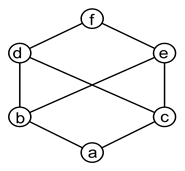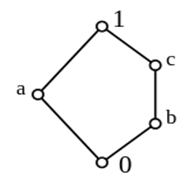# Welcome to the Graphs and Graphs Properties MCQs Page

Dive deep into the fascinating world of Graphs and Graphs Properties with our comprehensive set of Multiple-Choice Questions (MCQs). This page is dedicated to exploring the fundamental concepts and intricacies of Graphs and Graphs Properties, a crucial aspect of Discrete Mathematics. In this section, you will encounter a diverse range of MCQs that cover various aspects of Graphs and Graphs Properties, from the basic principles to advanced topics. Each question is thoughtfully crafted to challenge your knowledge and deepen your understanding of this critical subcategory within Discrete Mathematics.

Check out the MCQs below to embark on an enriching journey through Graphs and Graphs Properties. Test your knowledge, expand your horizons, and solidify your grasp on this vital area of Discrete Mathematics.

Note: Each MCQ comes with multiple answer choices. Select the most appropriate option and test your understanding of Graphs and Graphs Properties. You can click on an option to test your knowledge before viewing the solution for a MCQ. Happy learning!

### Graphs and Graphs Properties MCQs | Page 1 of 10

Q1.
The graph given below is an example of _________Q2.
The graph is the smallest non-modular lattice N₅. A lattice is _______ if and only if it does not have a _______ isomorphic to N₅.Q3.
A directed graph or digraph can have directed cycle in which ______
Answer: (b).starting node and ending node are same
Q4.
Let, D = <A, R> be a directed graph or digraph,then D’ = <A’, R’> is a subgraph if ___________
Answer: (a).A’ ⊂ A and R’ = R ∩ (A’ x A’)
Q5.
The graph representing universal relation is called _______
Q6.
What is a complete digraph?
Answer: (d).connection of every node with every other node including itself in a digraph
Q7.
Disconnected components can be created in case of ___________
Q8.
A simple graph can have _______
Answer: (d).no multiple edges, self-loops and parallel edges
Q9.
Degree of a graph with 12 vertices is _______